# Cell Data Types

Each cell in a worksheet has a value the CellValue object specifies. To access this object, use the CellRange.Value property. The data in a cell determines its value:

A cell’s value can be empty, numeric, text, Boolean or error, and can have various display formats. For example, a numeric value can be displayed as a decimal number, a percentage or currency, a date or time, etc.

Use the properties of the CellValue object the CellRange.Value property returns to retrieve information about the cell’s value type, and get the cell’s value as an object of the corresponding type. To get the string specifying the formatted value as it is displayed in a cell, use the Cell.DisplayText property.

The table below lists the available cell value types and provides examples of how to input, format, display and obtain these values.

Cell Value Type

Cell Content

Sample Input

Sample Display Format

Displayed String

Identify the Type

Obtain the Cell Value Object

Empty

The default cell value type.

If a cell contains any data, you can assign an empty value to it by setting the CellRange.Value property to null or CellValue.Empty.

cell.Value = null

• or -

cell.Value = CellValue.Empty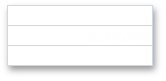CellValue.IsEmpty = true

CellValue.Empty

Numeric

The cell’s CellRange.Value property is assigned to a numeric type value (for example, Int32, Double, etc.).

• or -

The cell’s CellRange.Formula property is assigned to an expression that returns a number.

cell.Value = 12345678

• or -

cell.Formula = “= SUM(12000000,345678)”

cell.NumberFormat = “#,#”

More examples:

How to: Specify Number or Date Format for Cell Content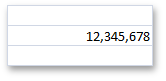CellValue.IsNumeric = true

CellValue.NumericValue

Numeric

(Date and Time)

The cell’s CellRange.Value property is assigned to the DateTime object the CellValue.FromDateTime method returns, or a numeric value representing a serial number of a date or time.

• or -

The cell’s CellRange.Formula property is assigned to an expression that returns the serial number of a date or time.

Refer to the Dates and Times in Cells document for more information.

cell.Value = new DateTime(2012, 12, 10);

• or -

workbook.DocumentSettings.Calculation.Use1904DateSystem = true;

cell.Value = CellValue.FromDateTime(new DateTime(2012, 12, 10), true);

• or -

cell.Value = 41253;

• or -

cell.Formula = “=DATE(2012,12,10)”;

cell.NumberFormat = “m/d/yy”

More examples:

How to: Specify Number or Date Format for Cell Content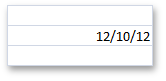CellValue.IsNumeric = true

CellValue.IsDateTime = true

CellValue.DateTimeValue

CellValue.NumericValue

Text

The cell’s CellRange.Value property is assigned to the String object.

• or -

The cell’s CellRange.Formula property is assigned to an expression that returns text.

cell.Value = “Sample Text”

• or -

cell.Formula = “= PROPER(“sample text”)”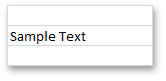CellValue.IsText = true

CellValue.TextValue

Boolean

The cell’s CellRange.Value property is assigned to the Boolean object.

• or -

The cell’s CellRange.Formula property is assigned to an expression that returns TRUE or FALSE.

cell.Value = true

• or -

cell.Formula = “= TRUE()”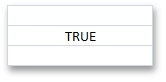CellValue.IsBoolean = true

CellValue.BooleanValue

Error

The cell’s CellRange.Value property is assigned to the CellValue object the CellValue.Error* field returns (for example, CellValue.ErrorDivisionByZero, CellValue.ErrorInvalidValueInFunction, CellValue.ErrorName, etc.).

• or -

The cell’s CellRange.Formula property is assigned to an error code (for example, “=#DIV/0!”, “=#N/A”, etc.) or an expression that cannot be calculated correctly (for example, an expression containing an invalid function name, value, division by zero, etc.)

cell.Value = CellValue.ErrorDivisionByZero

• or -

cell.Formula = “= #DIV/0!”

• or -

cell.Formula = “=5/0”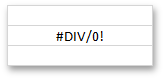CellValue.IsError = true

CellValue.ErrorValue